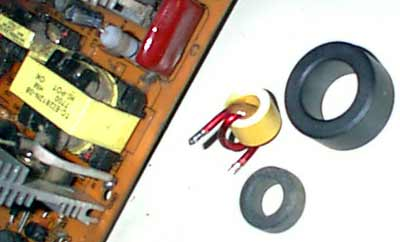# Calculating windings of pulse power transformer

In old magazine (Radio – Ð Ð°Ð´Ð¸Ð¾) I found small article about calculating PWM power transformers. The formulas are for toroid core transformers, but I think, they are usable in standard E core transformers too.

1. Calculate the power of transformer: P`t`=1.3*Î£P`n` , W. The power of transformer is sum of all output powers multiplied by 1.3.

2. The core max power: P=S`c`*S`o`*f*B`max`/150 , W. f=Hz, S`c`=area of core section in cm`2`, S`o`=area of the “hole” in cm`2`. B`max`= max induction in Teslas, from core datasheet.

3. Number of turns in primary winding: w`1`=0.25*10`4`*U`1`/fB`max`S`c` , in turns.

4. Max current in primary winding: I`1max`=P/Î·U`1` , A. (Î· = ~0.8)

5. Diameter of wire: d`1`=0.6*âˆšI`1max` , mm. diameter is equal 0.6 multiplied by square root of max current.

6. Secondary (ant other) winding: w`2`=w`1`*U`2`/U`1` , in turns.